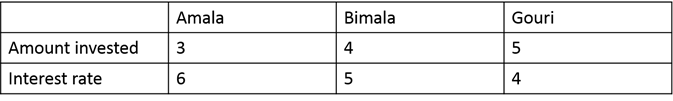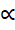# CAT 2019 Question Paper | Quants Slot 1

###### CAT Previous Year Paper | CAT Quants Questions | Question 23

Ratio and Proportion in its various avatars has been present in all CAT previous years paper. Here in CAT 2019, is a very simple ratio proportion question. As part of CAT Preparation, practice a lot of these questions to ensure you can maintain your speed and accuracy under pressure in the exam.

Question 23 : Amala, Bina, and Gouri invest money in the ratio 3 : 4 : 5 in fixed deposits having respective annual interest rates in the ratio 6 : 5 : 4. What is their total interest income (in Rs) after a year, if Bina's interest income exceeds Amala's by Rs 250?

1. 7000
2. 6000
3. 6350
4. 7250

## Best CAT Coaching in Chennai

#### CAT Coaching in Chennai - CAT 2022Limited Seats Available - Register Now!We know, Interest incomeAmount invested
And Interest incomeInterest rate
Therefore, Interest income must be in the ratio of the product of their Amount invested and Interest rate.
So, Ratios of Interest incomes of Amala, Bimala and Gouri = 18: 20: 20
Bina's Interest income exceeds Amala by 250 Rs.
So, 20 x - 18 x = 250
2x = 250
So, Total Interest income = 250 x (9 + 10 + 10) = 7250 Rs.
Total Interest Income = Rs. 7250

The question is "Amala, Bina, and Gouri invest money in the ratio 3 : 4 : 5 in fixed deposits having respective annual interest rates in the ratio 6 : 5 : 4. What is their total interest income (in Rs) after a year, if Bina's interest income exceeds Amala's by Rs 250? "

##### Hence, the answer is 7250

Choice D is the correct answer.

###### CAT Coaching in ChennaiCAT 2023

Classroom Batches Starting Now! @Gopalapuram

###### Best CAT Coaching in Chennai Introductory offer of 5000/-

Attend a Demo Class

##### Where is 2IIM located?

2IIM Online CAT Coaching
A Fermat Education Initiative,
58/16, Indira Gandhi Street,
Kaveri Rangan Nagar, Saligramam, Chennai 600 093

##### How to reach 2IIM?

Mobile: (91) 99626 48484 / 94459 38484
WhatsApp: WhatsApp Now
Email: info@2iim.com